# Wall group

An Abelian group associated with a ring with an involution which is an anti-isomorphism. In particular, it is defined for any group ring, where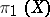is the fundamental group of a space. Ifis a Poincaré complex, then for a bordism classin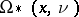there is an obstruction in this group to the existence of a simple homotopy equivalence in. This obstruction is called the Wall invariant, cf. .

Letbe a ring with an involutionwhich is an anti-isomorphism, i.e.. Ifis a left-module, then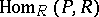is a left-module relative to the action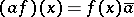,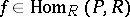,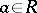,. This module is denoted by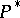. For a finitely-generated projective-modulethere is an isomorphism: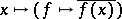, and one may identifyand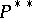using this isomorphism.

A quadratic-form over a ringwith an involution is a pair, whereis a finitely-generated projective-module andis a homomorphism such that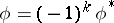. A morphismof forms is a homomorphismsuch that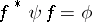. Ifis an isomorphism, then the form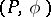is said to be non-degenerate. A Lagrange plane of a non-degenerate form is a direct summand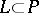for which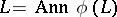. Ifis a direct summand such that, thenis called a subLagrange plane. Two Lagrange planes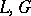of a formare called complementary ifand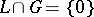.

Letbe a projective-module. The non-degenerate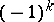-form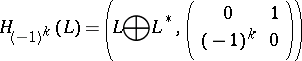is called Hamiltonian, and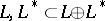are called its complementary Lagrange planes. Ifis a Lagrange plane of the form, then the form is isomorphic to the Hamiltonian form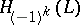. The choice of a Lagrange plane complementary tois equivalent to the choice of an isomorphism, and this complementary plane can be identified with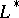.

Let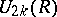be the Abelian group generated by the equivalence classes (under isomorphism) of non-degenerate quadratic-formswith the relations: 1)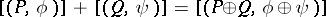; and 2)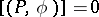ifhas a Lagrange plane. A tripleconsisting of a non-degenerate-formand a pair of Lagrange planes is called a-formation. A formation is said to be trivial ifandare complementary, and elementary if there exists a Lagrange plane ofwhich is complementary to bothand. The trivial formation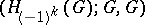is called Hamiltonian. By an isomorphism of formations,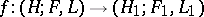, one understands an isomorphism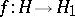of forms for which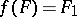,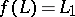. Every trivial formation is isomorphic to the Hamiltonian one.

Letbe the Abelian group generated by the equivalence classes (under isomorphism) of-formations with the following relations: a)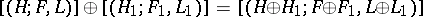; b)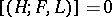if the formation is elementary or trivial.

The groupsare called the Wall groups of the ring.